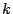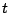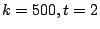An Extended Quadratic Frobenius Primality Test with Average Case Error Estimates Ivan Bjerre Damgård Gudmund Skovbjerg Frandsen November 2001

### Abstract:

We present an Extended Quadratic Frobenius Primality Test (EQFT), which is related to the Miller-Rabin test and the Quadratic Frobenius test (QFT) by Grantham. EQFT is well-suited for generating large, random prime numbers since on a random input number, it takes time about equivalent to 2 Miller-Rabin tests, but has much smaller error probability. EQFT extends QFT by verifying additional algebraic properties related to the existence of elements of order 3 and 4. We obtain a simple closed expression that upper bounds the probability of acceptance for any input number. This in turn allows us to give strong bounds on the average-case behaviour of the test: consider the algorithm that repeatedly chooses random oddbit numbers, subjects them toiterations of our test and outputs the first one found that passes all tests. We obtain numeric upper bounds for the error probability of this algorithm as well as a general closed expression bounding the error. For instance, it is at mostfor. Compared to earlier similar results for the Miller-Rabin test, the results indicates that our test in the average case has the effect of 9 Miller-Rabin tests, while only taking time equivalent to about 2 such tests. We also give bounds for the error in case a prime is sought by incremental search from a random starting point. While EQFT is slower than the average case on a small set of inputs, we present a variant that is always fast, i.e. takes time about 2 Miller-Rabin tests. The variant has slightly larger worst case error probability than EQFT, but still improves on previous proposed tests

Available as PostScript, PDF.